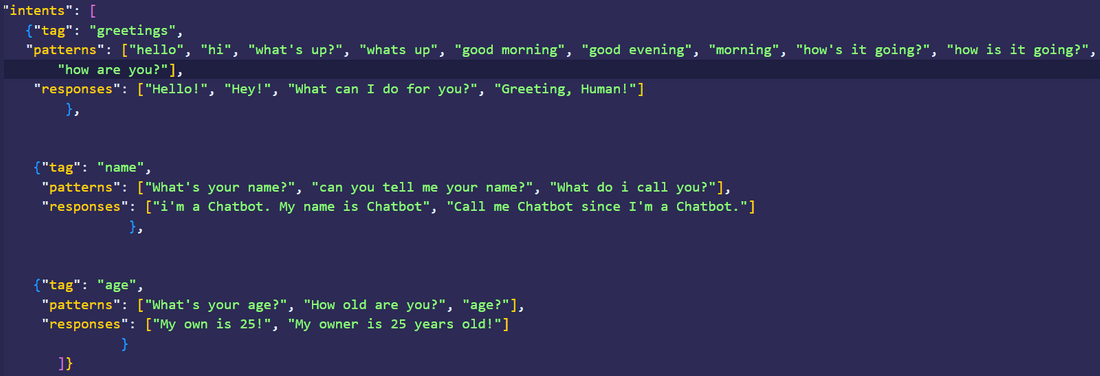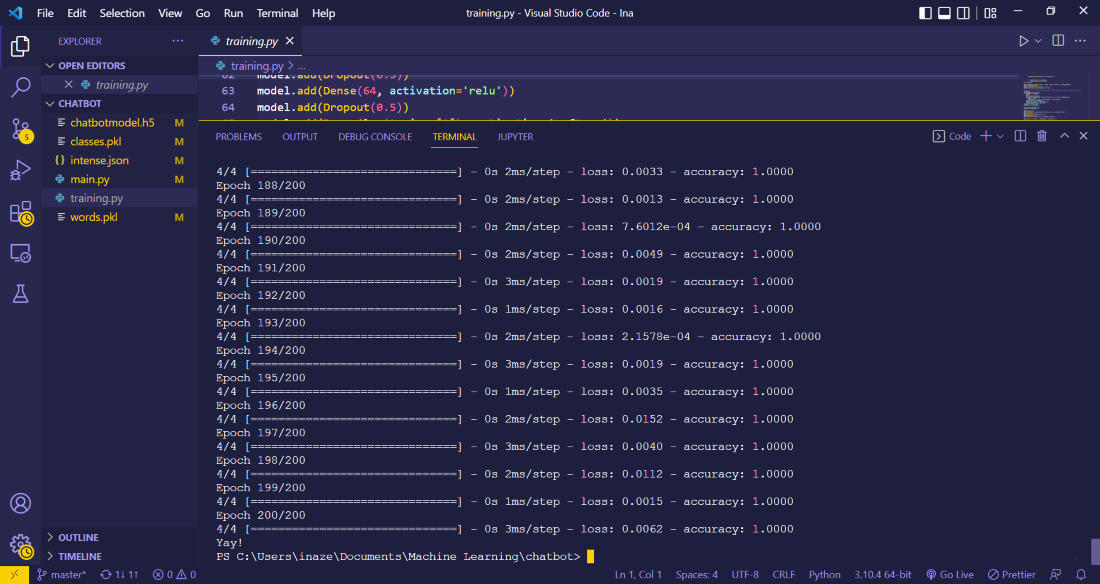# Deploy a Chatbot using TensorFlow in Python

• Last Updated : 02 Aug, 2022

In this article, you’ll learn how to deploy a Chatbot using Tensorflow. A Chatbot is basically a bot (a program) that talks and responds to various questions just like a human would. We’ll be using a number of Python modules to do this.

First, we’ll train the Chatbot model, and then in section two, we’ll learn how to make it work and respond to various inputs by the user.

## Modules required:

• random – This module is used to generate random responses from the Chatbot
• json – To read from json file
• pickle – To save data into files
• tensorflow – To train neural networks. It is an open source machine learning library.
• numpy – It is a Python library used for working with arrays
• nltk – It is a leading platform for building Python programs to work with human language data.

## Training the Chatbot model

The first thing we’re going to do is to train the Chatbot model. In order to do that, create a file named ‘intense.json’ in which we’ll write all the intents, tags and words or phrases our Chatbot would be responding to.

Step 1: Create a “intense.json”:

Here we’ve added just three tags just to show how it works. You can add a lot of them!Step 2: Create a “training.py”:

The next step is training our model.  We’ll use a class called WordNetLemmatizer() which will give the root words of the words that the Chatbot can recognize. For example, for hunting, hunter, hunts and hunted, the lemmatize function of the WordNetLemmatizer() class will give “hunt” because it is the root word.

• Create a WordNetLemmatizer() class object.
• Read the contents from the “intense.json” file and store it to a variable “intents”. Next, initialize empty lists to store the contents.
• Next up, we have a function called word_tokenize(para). It takes a sentence as a parameter and then returns a list containing all the words of the sentence as strings. Here we’re tokenizing the patterns and then appending them to a list ‘words’. So, at last, this list ‘words’ would have all the words that are in the ‘patterns’ list.
• In documents, we have all the patterns with their tags in the form of a tuple.
• Now, using a list comprehension, we’ll modify the list ‘words’ we created above and store the words’ ‘lemma’  or simply put, the root words.
• Dump the data of the ‘words’ and ‘classes’ to binary files of the same name, using the pickle module’s dump() function.

## Python3

 `# importing the required modules.``import` `random``import` `json``import` `pickle``import` `numpy as np``import` `nltk`` ` `from` `keras.models ``import` `Sequential``from` `nltk.stem ``import` `WordNetLemmatizer``from` `keras.layers ``import` `Dense, Activation, Dropout``from` `keras.optimizers ``import` `SGD`` ` ` ` `lemmatizer ``=` `WordNetLemmatizer()`` ` `# reading the json.intense file``intents ``=` `json.loads(``open``(``"intense.json"``).read())`` ` `# creating empty lists to store data``words ``=` `[]``classes ``=` `[]``documents ``=` `[]``ignore_letters ``=` `[``"?"``, ``"!"``, ``"."``, ``","``]``for` `intent ``in` `intents[``'intents'``]:``    ``for` `pattern ``in` `intent[``'patterns'``]:``        ``# separating words from patterns``        ``word_list ``=` `nltk.word_tokenize(pattern)``        ``words.extend(word_list)  ``# and adding them to words list``         ` `        ``# associating patterns with respective tags``        ``documents.append(((word_list), intent[``'tag'``]))`` ` `        ``# appending the tags to the class list``        ``if` `intent[``'tag'``] ``not` `in` `classes:``            ``classes.append(intent[``'tag'``])`` ` `# storing the root words or lemma``words ``=` `[lemmatizer.lemmatize(word)``         ``for` `word ``in` `words ``if` `word ``not` `in` `ignore_letters]``words ``=` `sorted``(``set``(words))`` ` `# saving the words and classes list to binary files``pickle.dump(words, ``open``(``'words.pkl'``, ``'wb'``))``pickle.dump(classes, ``open``(``'classes.pkl'``, ``'wb'``))`

Step 3: Now we need to classify our data into 0’s and 1’s because neural networks works with numerical values, not strings or anything else.

• Create an empty list called training, in which we’ll store the data used for training. Also create an output_empty list that will store as many 0’s as there are classes in the intense.json.
• Next up we’ll create a bag that will store the 0’s and 1’s. (0, if the word isn’t in the pattern and 1 if the word is in the pattern). To do that, we’ll iterate through the documents list and append 1 to the ‘bag’ if it is  not in the patterns, 0 otherwise.
• Now shuffle this training set and make it a numpy array.
• Split the training set consisting of 1’s and 0’s into two parts, that is train_x and train_y.

## Python3

 `# we need numerical values of the``# words because a neural network``# needs numerical values to work with``training ``=` `[]``output_empty ``=` `[``0``]``*``len``(classes)``for` `document ``in` `documents:``    ``bag ``=` `[]``    ``word_patterns ``=` `document[``0``]``    ``word_patterns ``=` `[lemmatizer.lemmatize(``        ``word.lower()) ``for` `word ``in` `word_patterns]``    ``for` `word ``in` `words:``        ``bag.append(``1``) ``if` `word ``in` `word_patterns ``else` `bag.append(``0``)``         ` `    ``# making a copy of the output_empty``    ``output_row ``=` `list``(output_empty)``    ``output_row[classes.index(document[``1``])] ``=` `1``    ``training.append([bag, output_row])``random.shuffle(training)``training ``=` `np.array(training)`` ` `# splitting the data``train_x ``=` `list``(training[:, ``0``])``train_y ``=` `list``(training[:, ``1``])`

Step 4: We’ve come to the model-building part of our Chatbot model. Here, we’re going to deploy a Sequential model, that we’ll train on the dataset we prepared above.

• Add():  This function is used to add layers in a neural network.
• Dropout(): This function is used to avoid overfitting

## Python3

 `# creating a Sequential machine learning model``model ``=` `Sequential()``model.add(Dense(``128``, input_shape``=``(``len``(train_x[``0``]), ),``                ``activation``=``'relu'``))``model.add(Dropout(``0.5``))``model.add(Dense(``64``, activation``=``'relu'``))``model.add(Dropout(``0.5``))``model.add(Dense(``len``(train_y[``0``]), ``                ``activation``=``'softmax'``))`` ` `# compiling the model``sgd ``=` `SGD(lr``=``0.01``, decay``=``1e``-``6``, momentum``=``0.9``, nesterov``=``True``)``model.``compile``(loss``=``'categorical_crossentropy'``,``              ``optimizer``=``sgd, metrics``=``[``'accuracy'``])``hist ``=` `model.fit(np.array(train_x), np.array(train_y),``                 ``epochs``=``200``, batch_size``=``5``, verbose``=``1``)`` ` `# saving the model``model.save(``"chatbotmodel.h5"``, hist)`` ` `# print statement to show the ``# successful training of the Chatbot model``print``(``"Yay!"``)`

Output:## Creating a main.py to run the Chatbot

We’re done training the model, now we need to create the main file that will make the Chatbot model work and respond to our inputs.

Step 1: To get started, import the following modules.

## Python3

 `# required modules``import` `random``import` `json``import` `pickle``import` `numpy as np``import` `nltk``from` `keras.models ``import` `load_model``from` `nltk.stem ``import` `WordNetLemmatizer`

Step 2: Initialize the following classes and file contents.

## Python3

 `lemmatizer ``=` `WordNetLemmatizer()`` ` `# loading the files we made previously``intents ``=` `json.loads(``open``(``"intense.json"``).read())``words ``=` `pickle.load(``open``(``'words.pkl'``, ``'rb'``))``classes ``=` `pickle.load(``open``(``'classes.pkl'``, ``'rb'``))``model ``=` `load_model(``'chatbotmodel.h5'``)`

Step 3: We’ll define 3 functions here.

clean_up_sentences(sentence) – This function will separate words from the sentences we’ll give as input.

## Python3

 `def` `clean_up_sentences(sentence):``    ``sentence_words ``=` `nltk.word_tokenize(sentence)``    ``sentence_words ``=` `[lemmatizer.lemmatize(word) ``                      ``for` `word ``in` `sentence_words]``    ``return` `sentence_words`

bagw(sentence): This function will append 1 to a list variable ‘bag’ if the word is contained inside our input and is also present in the list of words created earlier.

• First we’ll use the function defined above to separate out ‘root’ words from the input, then in the next line, initialise a list variable called bag that will contain as many 0’s as the length of the words list.
• Using nested for loops, we’ll check whether the word in the input is also in the words list. If it is, we’ll append 1 to the bag, otherwise it’ll remain 0.
• Return a numpy array of the list variable bag that now contains 1’s and 0’s.

## Python3

 `def` `bagw(sentence):``   ` `    ``# separate out words from the input sentence``    ``sentence_words ``=` `clean_up_sentences(sentence)``    ``bag ``=` `[``0``]``*``len``(words)``    ``for` `w ``in` `sentence_words:``        ``for` `i, word ``in` `enumerate``(words):`` ` `            ``# check whether the word``            ``# is present in the input as well``            ``if` `word ``=``=` `w:`` ` `                ``# as the list of words``                ``# created earlier.``                ``bag[i] ``=` `1`` ` `    ``# return a numpy array``    ``return` `np.array(bag)`

predict_class(sentence): This function will predict the class of the sentence input by the user.

• Initialize a variable bow that will contain a NumPy array of 0’s and 1’s, using the function defined above. Using the predict() function, we’ll predict the result based on the user’s input.
• Initialize a variable ERROR_THRESHOLD and append from ‘res’ if the value is greater than the ERROR_THRESHOLD, then sort it using the sort function.
• Using a list variable return_list, store the tag or classes that was in the intense.json file.

## Python3

 `def` `predict_class(sentence):``    ``bow ``=` `bagw(sentence)``    ``res ``=` `model.predict(np.array([bow]))[``0``]``    ``ERROR_THRESHOLD ``=` `0.25``    ``results ``=` `[[i, r] ``for` `i, r ``in` `enumerate``(res) ``               ``if` `r > ERROR_THRESHOLD]``    ``results.sort(key``=``lambda` `x: x[``1``], reverse``=``True``)``    ``return_list ``=` `[]``    ``for` `r ``in` `results:``        ``return_list.append({``'intent'``: classes[r[``0``]],``                            ``'probability'``: ``str``(r[``1``])})``        ``return` `return_list`

get_response(intents_list, intents_json): This function will print a random response from whichever class the sentence/words input by the user belongs to.

• First we initialize some required variables, such as tags, list_of_intents and results.
• If the tag matches the tags in the list_of_intents, store a random response in a variable called result, using the choice() method of the random module.
• Return result.

## Python3

 `def` `get_response(intents_list, intents_json):``    ``tag ``=` `intents_list[``0``][``'intent'``]``    ``list_of_intents ``=` `intents_json[``'intents'``]``    ``result ``=` `""``    ``for` `i ``in` `list_of_intents:``        ``if` `i[``'tag'``] ``=``=` `tag:``           ` `              ``# prints a random response``            ``result ``=` `random.choice(i[``'responses'``])  ``            ``break``    ``return` `result`` ` `print``(``"Chatbot is up!"``)`

Step 4: Finally, we’ll initialize an infinite while loop that will prompt the user for an input and print the Chatbot’s response.

## Python3

 `while` `True``:``    ``message ``=` `input``("")``    ``ints ``=` `predict_class(message)``    ``res ``=` `get_response(ints, intents)``    ``print``(res)`

main.py is as follows:

## Python3

 `import` `random``import` `json``import` `pickle``import` `numpy as np``import` `nltk``from` `keras.models ``import` `load_model``from` `nltk.stem ``import` `WordNetLemmatizer`` ` `lemmatizer ``=` `WordNetLemmatizer()``intents ``=` `json.loads(``open``(``"intense.json"``).read())``words ``=` `pickle.load(``open``(``'words.pkl'``, ``'rb'``))``classes ``=` `pickle.load(``open``(``'classes.pkl'``, ``'rb'``))``model ``=` `load_model(``'chatbotmodel.h5'``)`` ` `def` `clean_up_sentences(sentence):``    ``sentence_words ``=` `nltk.word_tokenize(sentence)``    ``sentence_words ``=` `[lemmatizer.lemmatize(word)``                      ``for` `word ``in` `sentence_words]``    ``return` `sentence_words`` ` `def` `bagw(sentence):``    ``sentence_words ``=` `clean_up_sentences(sentence)``    ``bag ``=` `[``0``]``*``len``(words)``    ``for` `w ``in` `sentence_words:``        ``for` `i, word ``in` `enumerate``(words):``            ``if` `word ``=``=` `w:``                ``bag[i] ``=` `1``    ``return` `np.array(bag)`` ` `def` `predict_class(sentence):``    ``bow ``=` `bagw(sentence)``    ``res ``=` `model.predict(np.array([bow]))[``0``]``    ``ERROR_THRESHOLD ``=` `0.25``    ``results ``=` `[[i, r] ``for` `i, r ``in` `enumerate``(res)``               ``if` `r > ERROR_THRESHOLD]``    ``results.sort(key``=``lambda` `x: x[``1``], reverse``=``True``)``    ``return_list ``=` `[]``    ``for` `r ``in` `results:``        ``return_list.append({``'intent'``: classes[r[``0``]],``                            ``'probability'``: ``str``(r[``1``])})``        ``return` `return_list`` ` `def` `get_response(intents_list, intents_json):``    ``tag ``=` `intents_list[``0``][``'intent'``]``    ``list_of_intents ``=` `intents_json[``'intents'``]``    ``result ``=` `""``    ``for` `i ``in` `list_of_intents:``        ``if` `i[``'tag'``] ``=``=` `tag:``            ``result ``=` `random.choice(i[``'responses'``])``            ``break``    ``return` `result`` ` `print``(``"Chatbot is up!"``)`` ` `while` `True``:``    ``message ``=` `input``("")``    ``ints ``=` `predict_class(message)``    ``res ``=` `get_response(ints, intents)``    ``print``(res)`

Output:

My Personal Notes arrow_drop_up